An electric dipole is a pair of equal and opposite charges q and –q separated by some distance 2a. Its dipole moment vector p has magnitude 2qa and is in the direction of the dipole axis from –q to q.

### The field of an electric dipole

The electric field of the pair of charges (–q and q) at any point in space can be found out from Coulomb’s law and the superposition principle.

The results are simple for the following two cases:

• When the point is on the dipole axis
• When it is in the equatorial plane of the dipole, i.e., on a plane perpendicular to the dipole axis through its center.

### 1. For Points on the axis

Let the point P be at distance r from the center of the dipole on the side of the charge q, as shown in Fig.(a). Then,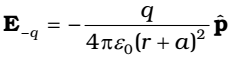Where is the unit vector along the dipole axis (from –q to q). Also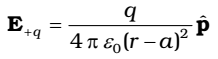The total field at P is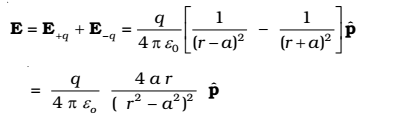For r ≫ a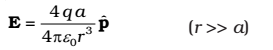### 2. For points on the equatorial plane

The magnitudes of the electric fields due to the two charges +q and –q are equal.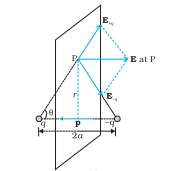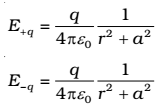The directions of E+q and E-q are shown in fig.

The total electric field is opposite to .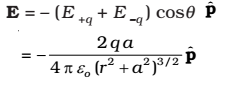At large distance (r ≫ a), this reduces to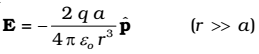It is clear that the dipole field at large distances does not involve q and a separately; it depends on the product q a. This suggests the definition of dipole moment. The dipole moment vector p of an electric dipole is defined by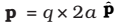At a point on the dipole axis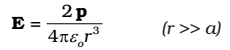At a point on the equatorial plane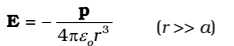The important point that the dipole field at large distances falls off not as 1/r2 but as1/r3. Further, the magnitude and the direction of the dipole field depend not only on the distance r but also on the angle between the position vector r and the dipole moment p.

### 3. Dipole in a Uniform External Field

There is a force qE on q and a force –qE on –q. The net force on the dipole is zero since E is uniform. However, the charges are separated, so the forces act at different points, resulting in a torque on the dipole. When the net force is zero, the torque (couple) is independent of the origin. Its magnitude equals the magnitude of each force multiplied by the arm of the couple (perpendicular distance between the two anti-parallel forces).Dipole in a uniform electric field

Magnitude of torque = q E × 2a sinθ

= 2 q a E sinθ

Its direction is normal to the plane of the paper, coming out of it. The magnitude of p × E is also p E sinθ and its direction is normal to the paper, coming out of it. Thus,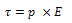This torque will tend to align the dipole with the field E. when p is aligned with W, the torque is zero.